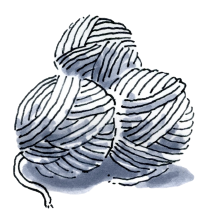### Home > CC1 > Chapter 6 > Lesson 6.1.3 > Problem6-39

6-39.

Jesse has five meters of twine and needs to cut it into lengths that are each $\frac { 1 } { 4 }$ of a meter long. How many lengths will he have? Express this problem in a number sentence that uses division.

There are $4$ fourths in $1$. How many fourths are there in $5$?

$\text{This can be written as }5\div \frac{1}{4}.$